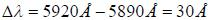Courses

# Test: Classical Mechanics - 2

## 20 Questions MCQ Test GATE Physics Mock Test Series | Test: Classical Mechanics - 2

Description
This mock test of Test: Classical Mechanics - 2 for GATE helps you for every GATE entrance exam. This contains 20 Multiple Choice Questions for GATE Test: Classical Mechanics - 2 (mcq) to study with solutions a complete question bank. The solved questions answers in this Test: Classical Mechanics - 2 quiz give you a good mix of easy questions and tough questions. GATE students definitely take this Test: Classical Mechanics - 2 exercise for a better result in the exam. You can find other Test: Classical Mechanics - 2 extra questions, long questions & short questions for GATE on EduRev as well by searching above.
QUESTION: 1

### For a system with one degree of freedom, hamiltonian is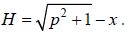The shape of x-t graph for the particle is

Solution: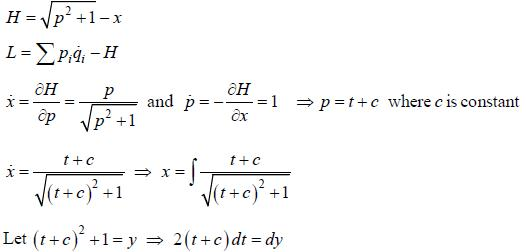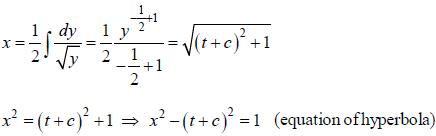QUESTION: 2

### A particle of charge q and rest mass m0 is kept at rest at the origin. A constant uniform electric field,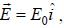starts acting on it at t = 0. The speed of the particle at time t is (in the following c is speed of light)

Solution: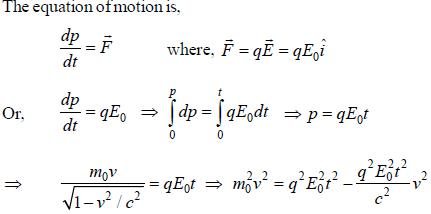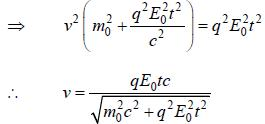QUESTION: 3

### The Lagrangian of a system is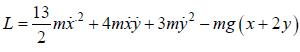Which one of the following is conserved?

Solution: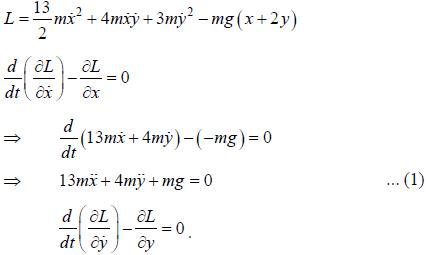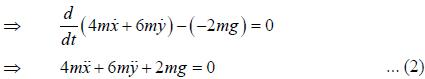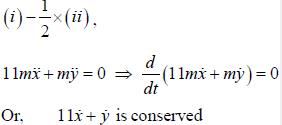QUESTION: 4

A pendulum consists of a mass m and a massless stick of length l. The pendulum support oscillates horizontally with a position given by x (t) =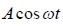(see figure). The momentum conjugate to θ is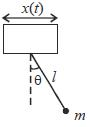Solution:

The position of mass m is given by,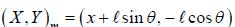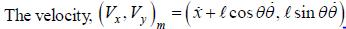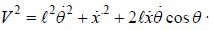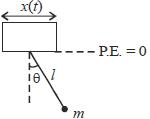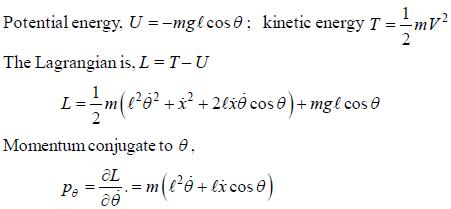QUESTION: 5

If the Hamiltonian of a system with two degrees of freedom is given by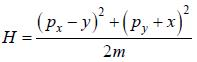then the quantity that is conserved is

Solution: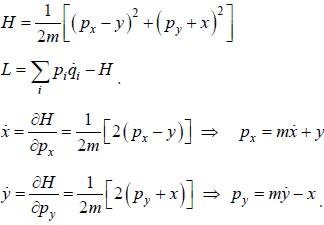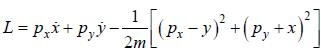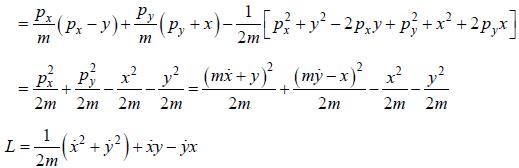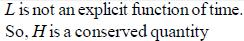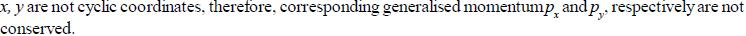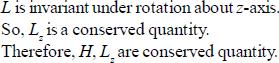QUESTION: 6

The Poisson bracket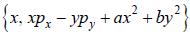with a and b as constants is equal to

Solution: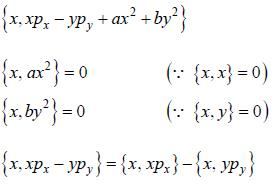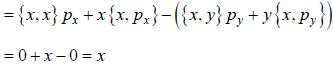QUESTION: 7

A particle of effective mass m is acted on by a potential energy given by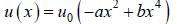where, u0, a and b are positive constants, then

Solution: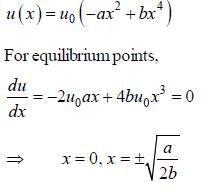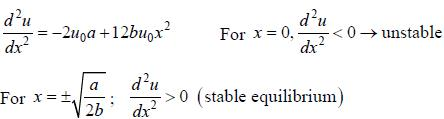*Answer can only contain numeric values
QUESTION: 8

The number of degrees of freedom of two particles moving on a space curve and having constant distance between them, is ______.

Solution:

Degree of freedom (D.O.F.) of each particle on a curve = 1
D.O.F. of two particles = 2x1 =2
Number of constraints = 1 (constant distance)
Therefore. D.O.F. of the system = 2-1 = 1

*Answer can only contain numeric values
QUESTION: 9

The average lifetime of a π -meson in its own frame of reference is 26.011s. If the π - meson moves with speed 0.95c with respect to the earth, then the average distance it travels before decaying as measured byan observer at rest on Earth is approximately close to _______________________ meters (nearest integer)

Solution:

Proper lifetime, =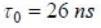Lifetime measured by observer on earth.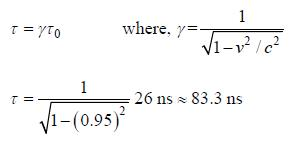The average distance it travels before decaying as measured byan observer at rest on Earth.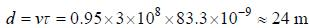*Answer can only contain numeric values
QUESTION: 10

A particle of mass m is subjected to a central force given by the potential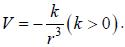The radius of the circular orbit for a particle with angular momentum,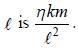The value of η is ______.

Solution: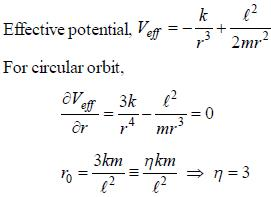QUESTION: 11

The path of a particle of mass m moving minder some potential V ( r ) is given by the r = A exp (aθ). where A and a are constants. The form of V(r) is (in the following L and E are angular momentum, and total energy, respectively)

Solution: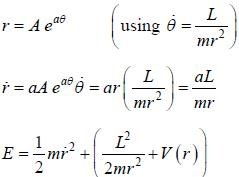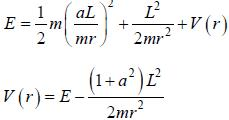QUESTION: 12

A system with one degree of freedom has Lagrangian,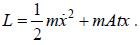The equation of x(t) under the initial conditions t = 0, x = 0, p = mv0, is

Solution: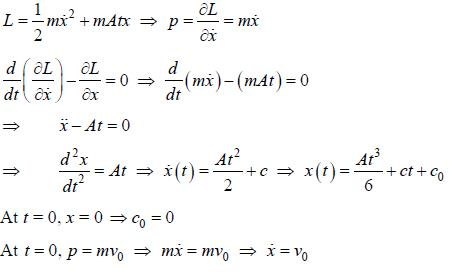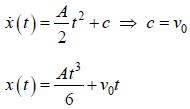QUESTION: 13

The dynamics of a particle moving on the real line is determined by the Hamiltonian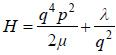where μ and λ are constants. Then the value of dp/dq is

Solution: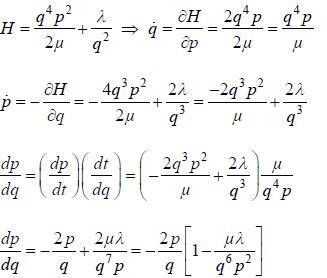QUESTION: 14

Hamiltonian of a one dimensional dynamical system is given by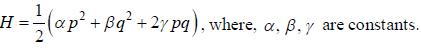The Poisson bracket {p, H} is equal to

Solution: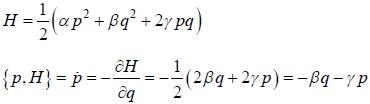QUESTION: 15

For a charged particle of mass m and charge e in a magnetic field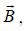the Lagrangian is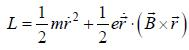The corresponding Hamiltonian is

Solution: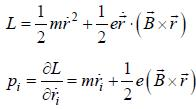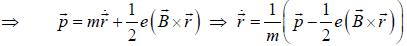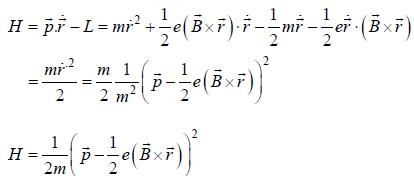*Answer can only contain numeric values
QUESTION: 16

Given that the linear transformation of a generalized coordinate q and corresponding momentum p,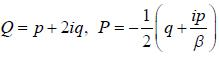is canonical, the value of the constant β is ______ (Here, i = √-1)

Solution: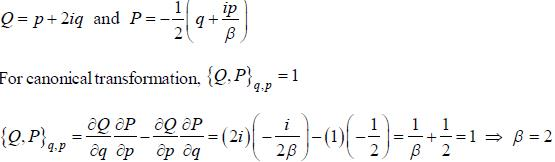*Answer can only contain numeric values
QUESTION: 17

Two events in a inertial referance frame separated by a distance 3.6 x 108m and occur 2s apart. The proper time interval between the occurrence of these two events is _____ sec. (upto one decimal place)

Solution:

Consider an inertial reference frams S' moving relative to the given frame S (in equation) where the two events occurs at same spatial location,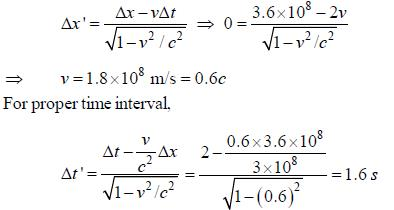*Answer can only contain numeric values
QUESTION: 18

The moment of inertia of a square frame of side length a and mass M about an axis passing through one of its
comers and perpendicular to its plane is βMa2. The value of β is________(upto two decimal places)

Solution:

The mass of the square frame is M
The sides ot square frame can be treated as rods ot mass 3/4 and length a.
Moment of inertia of one rod about an axis passing through its centre and perpendicular to its plane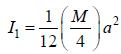Using parallel axis theorem, moment of inertia of the rod about an axis passing through centre of square frame and perpendicular to its plane.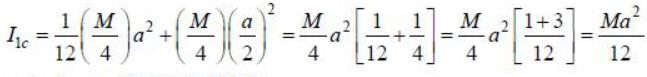By symmetry clue to all rock, total M.I.,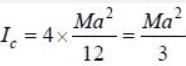Now, using parallel axis theorem again, to find moment of inertia about the axis given in question,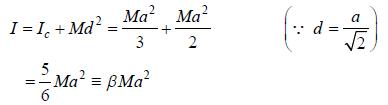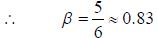*Answer can only contain numeric values
QUESTION: 19

Ail object of mass m = 100 kg and volume V= 0.18 m3 is dropped from a height h = 100m above the surface of the water. Upon entering water (β= 1000 kg/m3 ), the object experiences a resistance force given by F = -kv. where k = 10N sec in. The maximum depth to which the object will sink before starting to rise again is _____ m. (Neglect air resistance and assume the object looses negligible energy when going through the surface of the water). (Nearest integer)

Solution:

Let the velocity with which the object hits the water be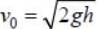The equation of motion after it enters the water.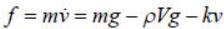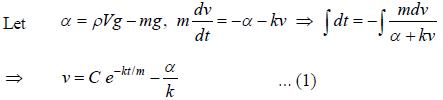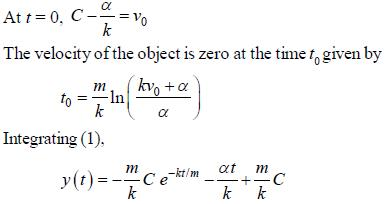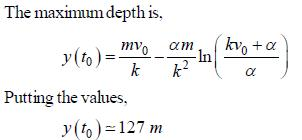*Answer can only contain numeric values
QUESTION: 20

A star receding from the earth at a speed of 5 x 10-3c. The wavelength shift for the sodium D2 line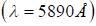is approximately equal to ____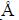(nearest integer)

Solution:

The Doppler equation gives,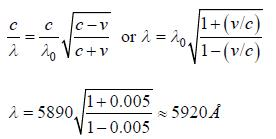Wavelength shift,